## Variance-Covariance Matrix¶

Miftahur Rahman, Ph.D.
March 26, 2022

The motivation of writing a quick review article on "$\bf Variance-Covariance \ Matrix$" is to study the protection of active satellites and International Space Station (ISS) from impacts by space debris objects. Collision avoidance for the orbiting satellites and ISS serves both adaptation and mitigation. A major collision such as the Iridium-Cosmos collision in early 2009 created much additional debris and thus, as the debris population increases collision avoidance becomes urgently necessary. The Joint Space Operations Center of the U.S. conducting conjunction assessments for all operational spacecraft in Earth's orbits, regardless of ownership. Any prediction of a close approach, typically within 1 km, will be notified with the spacecraft owner or the operator immediately without any cost.

Predictions of the collision in between of the satellites, satellite and debris are probabilistic due to inherent uncertainties in space surveillance measurements, the dynamic state of the atmosphere and, the instability of at least one of the conjuncting objects. Typical maneuver probability thresholds for collision avoidance are 1 in 10,000 for human space flight and 1 in 1000 (or more) for robotic satellites.

Collision avoidance maneuvers normally integrate well with standard satellite operations. Collision avoidance maneuvers are typically very small, that is, involve changes in velocity of less than $1\frac{m}{s}$$1 \frac {m}{s}$ and in most cases can be conducted in a manner that does not waste propellant resources. As an example, collision avoidance maneuvers performed by the ISS and robotic satellites are almost always result in a small increase in orbital altitude and thus simply constitute an unscheduled antidrag maneuver.

More than 99% of the risk to operational spacecraft comes from collisions with objects too small to track routinely, that is, objects smaller than 5–10 cm. Therefore, there is no alternative to an improvement in the orbital debris environment that could drastically reduce collision risks for operational spacecraft.

Covariance matrices, are compact summaries of the variability and covariation present in data. The covariance matrix and vector of means constitute sufficient statistics for models that assume a multivariate normal distribution.

$\mathbf{V}\mathbf{a}\mathbf{r}\mathbf{i}\mathbf{a}\mathbf{n}\mathbf{c}\mathbf{e}$$\bf Variance$ is a measure of the variability or spread in a set of data. Mathematically, it is the average squared deviation from the mean data. We use the following formula to compute population variance.

${\sigma }_{x}^{2}=Var\left(X\right)=\sum _{i=0}^{N}\frac{\left({X}_{i}-\overline{X}{\right)}^{2}}{N}=\sum \frac{{x}_{i}^{2}}{N}$

where

N is the total number of data points in a data set,
$\overline{X}$$\bar X$ is the mean of the N number of data set,
${X}_{i}$$X_i$ is the ith raw data in the set of data,
${x}_{i}$$x_i$ is the ith deviation of data in the set of data,
Var(X) is the variance of all the data points in the data set.

$\mathbf{C}\mathbf{o}\mathbf{v}\mathbf{a}\mathbf{r}\mathbf{i}\mathbf{a}\mathbf{n}\mathbf{c}\mathbf{e}$$\bf Covariance$ is a measure of the extent to which corresponding elements from two sets of ordered data move in the same direction. We use the following formula to compute population covariance.

$\sigma \left(x,y\right)=Cov\left(X,Y\right)=\sum \frac{\left({X}_{i}-\overline{X}\right)\left({Y}_{i}-\overline{Y}\right)}{N}=\sum \frac{{x}_{i}{y}_{i}}{N}$

where

N is the number of data points in each set of data,
$\overline{X}$$\bar X$ is the mean of the N data points in the first data set,
${X}_{i}$$X_i$ is the ith raw data in the first set of data,
${x}_{i}$$x_i$ is the ith deviation of data in the first set of data,
$\overline{Y}$$\bar Y$ is the mean of the N data in the second data set,
${Y}_{i}$$Y_i$ is the ith raw data in the second set of data,
${y}_{i}$$y_i$ is the ith deviation data in the second set of data,
Cov(X,Y) is the covariance of corresponding data in the two sets of data.

### Variance-Covariance Matrix¶

$\bf Variance \ and \ covariance$ are often displayed together in a variance-covariance matrix. The variances appear along the diagonal and covariances appear in the off-diagonal elements, as shown below.

$\mathbf{V}\mathbf{=}\left[\begin{array}{ccc}\sum \frac{{x}_{1}^{2}}{N}& \sum \frac{{x}_{1}{x}_{2}}{N}& .....\sum \frac{{x}_{1}{x}_{r}}{N}\\ \sum \frac{{x}_{2}{x}_{1}}{N}& \sum \frac{{x}_{2}^{2}}{N}& .....\sum \frac{{x}_{2}{x}_{r}}{N}\\ .....\\ \sum \frac{{x}_{r}{x}_{1}}{N}& \sum \frac{{x}_{r}{x}_{2}}{N}& .....\sum \frac{{x}_{r}^{2}}{N}\end{array}\right]$

where

$\mathbf{V}$$\bf V$ is a r x r variance-covariance matrix,
N is the number of data points in each of the r data sets,
${x}_{i}$$x_i$ is a deviation data from the ith data set,
$\sum \frac{{x}_{i}^{2}}{N}$$\sum \frac{x_i^2}{N}$ is the variance of elements from the ith data set,
$\sum \frac{{x}_{i}{x}_{j}}{N}$$\sum \frac{x_ix_j}{N}$ is the covariance for elements from the ith and jth data sets.

### Example of Variance-Covariance Matrix¶

Suppose $\mathbf{A}$$\bf A$ is an n x k matrix holding ordered sets of raw data of the scores on k tests for n students, as shown in Example-1.

Starting with the raw data of matrix $\mathbf{A}$$\bf A$, you can create a variance-covariance matrix to show the variance within each column and the covariance between columns. Here's how.

Transform the raw scores from matrix X into deviation scores for matrix x.

$\mathbf{a}$$\bf a$ = $\mathbf{A}$$\bf A$ - $\mathbf{1}$$\bf 1$ ${\mathbf{1}}^{\prime }$$\bf 1'$ $\mathbf{A}$$\bf A$ ( 1 / n )

where

1 is an n x 1 column vector of ones 1' is the transpose of vector 1
$\mathbf{a}$$\bf a$ is an n x k matrix of deviation scores: ${a}_{11}$$a_{11}$, ${a}_{12}$$a_{12}$, . . . , ${a}_{nk}$$a_{nk}$
$\mathbf{A}$$\bf A$ is an n x k matrix of raw scores: ${A}_{11}$$A_{11}$, ${A}_{12}$$A_{12}$, . . . , ${A}_{nk}$$A_{nk}$

Compute ${\mathbf{a}}^{\prime }\mathbf{a}$$\bf a'a$, the k x k deviation sums of squares and cross products matrix for $\mathbf{a}$$\bf a$.

Then, divide each term in the deviation sums of squares and cross product matrix by n to create the variance-covariance matrix. That is,

$\mathbf{V}\mathbf{=}{\mathbf{a}}^{\prime }\mathbf{a}\mathbf{\left(}\mathbf{1}\mathbf{/}\mathbf{n}\mathbf{\right)}$

where

$\mathbf{V}$$\bf V$ is a k x k variance-covariance matrix, ${\mathbf{a}}^{\prime }$$\bf a'$ is the transpose of matrix $\mathbf{a}$$\bf a$, ${\mathbf{a}}^{\prime }\mathbf{a}$$\bf a'a$ is the deviation sums of squares and cross product matrix, n is the number of scores in each column of the original matrix $\mathbf{A}$$\bf A$,

### Example-1¶

The table below displays scores on PHY, MAT, and ENG tests for 5 students.

 Student PHY MAT ENG 1 90 60 90 2 90 90 30 3 60 60 60 4 60 60 90 5 30 30 30

Note that data from the table can be represented in matrix $\mathbf{A}$$\bf A$, where each column in the matrix shows scores on a test and each row shows scores for a student.

$\mathbf{A}\mathbf{=}\left[\begin{array}{ccc}90& 60& 90\\ 90& 90& 30\\ 60& 60& 60\\ 60& 60& 90\\ 30& 30& 30\end{array}\right]$

Given the data represented in matrix $\mathbf{A}$$\bf A$, compute the variance of each test and the covariance between the tests.

The solution involves a three-step process.

First, we transform the raw scores in matrix $\mathbf{A}$$\bf A$ to deviation scores in matrix $\mathbf{a}$$\bf a$, using the transformation formula described at how to transform raw scores to deviation scores.

$\mathbf{a}\mathbf{=}\mathbf{A}\mathbf{-}{\mathbf{11}}^{\prime }\mathbf{A}\left(\frac{\mathbf{1}}{\mathbf{n}}\right)$

where

1 is an 5 x 1 column vector of ones,
$\mathbf{a}$$\bf a$ is an 5 x 3 matrix of deviation scores: ${a}_{11}$$a_{11}$, ${a}_{12}$$a_{12}$, . . . , ${a}_{53}$$a_{53}$
$\mathbf{A}$$\bf A$ is an 5 x 3 matrix of raw scores: ${A}_{11}$$A_{11}$, ${A}_{12}$$A_{12}$, . . . , ${A}_{53}$$A_{53}$
n is the number of rows in matrix $\mathbf{A}$$\bf A$

$\mathbf{a}\mathbf{=}\left[\begin{array}{ccc}90& 60& 90\\ 90& 90& 30\\ 60& 60& 60\\ 60& 60& 90\\ 30& 30& 30\end{array}\right]\mathbf{-}\left[\begin{array}{ccccc}1& 1& 1& 1& 1\\ 1& 1& 1& 1& 1\\ 1& 1& 1& 1& 1\\ 1& 1& 1& 1& 1\\ 1& 1& 1& 1& 1\end{array}\right]\left[\begin{array}{ccc}90& 60& 90\\ 90& 90& 30\\ 60& 60& 60\\ 60& 60& 90\\ 30& 30& 30\end{array}\right]\left(\frac{\mathbf{1}}{\mathbf{5}}\right)$
$\mathbf{a}\mathbf{=}\left[\begin{array}{ccc}90& 60& 90\\ 90& 90& 30\\ 60& 60& 60\\ 60& 60& 90\\ 30& 30& 30\end{array}\right]\mathbf{-}\left[\begin{array}{ccc}66& 60& 60\\ 66& 60& 60\\ 66& 60& 60\\ 66& 60& 60\\ 66& 60& 60\end{array}\right]$
$\mathbf{a}\mathbf{=}\left[\begin{array}{ccc}24& 0& 30\\ 24& 30& -30\\ -6& 0& 0\\ -6& 0& 30\\ -36& -30& -30\end{array}\right]$

Then, to find the deviation score sums of squares matrix, we compute a'a, as shown below.

${\mathbf{a}}^{\prime }\mathbf{a}\mathbf{=}\left[\begin{array}{ccccc}24& 24& -6& -6& -36\\ 0& 30& 0& 0& -30\\ 30& -30& 0& 30& -30\end{array}\right]\mathbf{-}\left[\begin{array}{ccc}66& 60& 60\\ 66& 60& 60\\ 66& 60& 60\\ 66& 60& 60\\ 66& 60& 60\end{array}\right]$
${\mathbf{a}}^{\prime }\mathbf{a}\mathbf{=}\left[\begin{array}{ccc}2520& 1800& 900\\ 1800& 1800& 0\\ 900& 0& 3600\end{array}\right]$

And finally, to create the variance-covariance matrix, we divide each element in the deviation sum of squares matrix by n, as shown below.

$\mathbf{V}\mathbf{=}\frac{{\mathbf{a}}^{\prime }\mathbf{a}}{\mathbf{5}}\mathbf{=}\left[\begin{array}{ccc}2520& 1800& 900\\ 1800& 1800& 0\\ 900& 0& 3600\end{array}\right]\left(\frac{\mathbf{1}}{\mathbf{5}}\right)$
$\mathbf{V}\mathbf{=}\left[\begin{array}{ccc}504& 360& 180\\ 360& 360& 0\\ 180& 0& 720\end{array}\right]$

We can interpret the variance and covariance statistics in matrix $\mathbf{V}$$\bf V$ to understand how the various test scores vary and covary.

Shown along the diagonal, we see the variance of scores for each test. The English test has the biggest variance (720); and the MAT test, the smallest (360). So we can say that English test scores are more variable than MAT test scores. The covariance is displayed in the off-diagonal elements of matrix $\mathbf{V}$$\bf V$.

The covariance between PHY and MAT is positive (360), and the covariance between PHY and ENG is positive (180). This means the scores tend to covary in a positive way. As scores on PHY go up, scores on ENG and MAT also tend to go up; and vice versa.

The covariance between MAT and ENG, however, is zero. This means there tends to be no predictable relationship between the movement of MAT and ENG scores. If the covariance between any tests had been negative, it would have meant that the test scores on those tests tend to move in opposite directions. That is, students with relatively high scores on the first test would tend to have relatively low scores on the second test.

### Steps to Create a Covariance Matrix using Python¶

Now we are going to test the Example-1 using Python libraries.

### Step 1: Using the Same Dataset¶

 Student PHY MAT ENG 1 90 60 90 2 90 90 30 3 60 60 60 4 60 60 90 5 30 30 30

### Step 2: Get the Population Covariance Matrix using Python¶

To get the population covariance matrix (based on N), you’ll need to set the bias to True in the code below.

This is the complete Python code to derive the population covariance matrix using the numpy package:

In :
import numpy as np

PHY = [90,90,60,60,30]
MAT = [60,90,60,60,30]
ENG = [90,30,60,90,30]

data = np.array([PHY,MAT,ENG])

covMatrix = np.cov(data,bias=True)
print (covMatrix)

[[504. 360. 180.]
[360. 360.   0.]
[180.   0. 720.]]


### Step 3: Get a Visual Representation of the Matrix¶

You can use the seaborn and matplotlib packages in order to visually represent the covariance matrix.

Here is the complete code that you can apply in Python:

In :
import numpy as np
import seaborn as sn
import matplotlib.pyplot as plt

PHY = [90,90,60,60,30]
MAT = [60,90,60,60,30]
ENG = [90,30,60,90,30]

data = np.array([PHY,MAT,ENG])

covMatrix = np.cov(data,bias=True)
sn.heatmap(covMatrix, annot=True, fmt='g')
plt.show()

<Figure size 640x480 with 2 Axes>

### Derive the Sample Covariance Matrix¶

To get the sample covariance (based on N-1), you’ll need to set the bias to False in the code below.

Here is the code based on the numpy package:

In :
import numpy as np

PHY = [90,90,60,60,30]
MAT = [60,90,60,60,30]
ENG = [90,30,60,90,30]

data = np.array([PHY,MAT,ENG])

covMatrix = np.cov(data,bias=False)
print (covMatrix)

[[630. 450. 225.]
[450. 450.   0.]
[225.   0. 900.]]


You can also use the pandas package in order to get the sample covariance matrix.

You may then apply the following code using pandas:

In :
import pandas as pd

data = {'PHY': [90,90,60,60,30],
'MAT': [60,90,60,60,30],
'ENG': [90,30,60,90,30]
}

df = pd.DataFrame(data,columns=['PHY','MAT','ENG'])

covMatrix = pd.DataFrame.cov(df)
print (covMatrix)

       PHY    MAT    ENG
PHY  630.0  450.0  225.0
MAT  450.0  450.0    0.0
ENG  225.0    0.0  900.0


Finally, you can visually represent the covariance matrix using the seaborn and matplotlib packages:

In :
import pandas as pd
import seaborn as sn
import matplotlib.pyplot as plt

data = {'PHY': [90,90,60,60,30],
'MAT': [60,90,60,60,30],
'ENG': [90,30,60,90,30]
}
df = pd.DataFrame(data,columns=['PHY','MAT','ENG'])

covMatrix = pd.DataFrame.cov(df)
sn.heatmap(covMatrix, annot=True, fmt='g')
plt.show()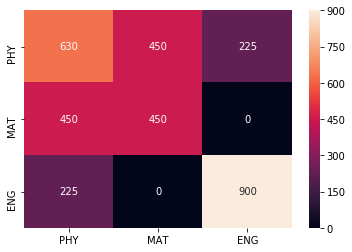### Geometric and Intuitive Explanation¶

Following is a geometric and intuitive explanation of the covariance matrix and the way it describes the shape of a data set. We will describe the geometric relationship of the covariance matrix with the use of linear transformations and eigendecomposition.

Before we get started, we shall take a quick look at the difference between covariance and variance. Variance measures the variation of a single random variable (like the height of a person in a population), whereas covariance is a measure of how much two random variables vary together (like the height of a person and the weight of a person in a population). The formula for variance is given by

${\sigma }_{x}^{2}=\frac{1}{n-1}\sum _{i=1}^{n}\left({x}_{i}-\overline{x}{\right)}^{2}$

where n is the number of samples (e.g. the number of people) and $\overline{x}$$\bar x$ is the mean of the random variable $\mathbf{x}$$\bf x$ (represented as a vector). The covariance $\sigma \left(x,y\right)$$\sigma (x,y)$ of two random variables x and y is given by

$\sigma \left(x,y\right)=\frac{1}{n-1}\sum _{i=1}^{n}\left({x}_{i}-\overline{x}\right)\left({y}_{i}-\overline{y}\right)$

with n samples. The variance ${\sigma }_{x}^{2}$$\sigma_x^2$ of a random variable x can be also expressed as the covariance with itself by $\sigma \left(x,x\right)$$\sigma(x,x)$.

### Covariance Matrix¶

With the covariance we can calculate entries of the covariance matrix, which is a square matrix given by

${C}_{i,j}=\sigma \left({x}_{i},{x}_{j}\right)$$C_{i,j}=\sigma(x_i,x_j)$ where $C\in {\mathbb{R}}^{d×d}$$C \in \mathbb{R}^{d \times d}$ and d describes the dimension or number of random variables of the data (e.g. the number of features like height, width, weight, …). Also the covariance matrix is symmetric since $\sigma \left({x}_{i},{x}_{j}\right)=\sigma \left({x}_{j},{x}_{i}\right)$$\sigma(x_i,x_j) = \sigma(x_j,x_i)$. The diagonal entries of the covariance matrix are the variances and the other entries are the covariances. For this reason, the covariance matrix is sometimes called the variance-covariance matrix. The calculation for the covariance matrix can be also expressed as

$C=\frac{1}{n-1}\sum _{i=1}^{n}\left({X}_{i}-\overline{X}\right)\left({X}_{i}-\overline{X}{\right)}^{T}$

where our data set is expressed by the matrix $X\in {\mathbb{R}}^{n×d}$$X \in \mathbb{R}^{n \times d}$. Following from this equation, the covariance matrix can be computed for a data set with zero mean with $C=\frac{X{X}^{T}}{n-1}$$C = \frac{XX^T}{n-1}$ by using the semi-definite matrix $X{X}^{T}$$XX^T$.

In this article, we will focus on the two-dimensional case, but it can be easily generalized to more dimensional data. Following from the previous equations the covariance matrix for two dimensions is given by

$C=\left[\begin{array}{cc}\sigma \left(x,x\right)& \sigma \left(x,y\right)\\ \sigma \left(y,x\right)& \sigma \left(y,y\right)\end{array}\right]$

We want to show how linear transformations affect the data set and in result the covariance matrix. First we will generate random points with mean values $\overline{x},\overline{y}$$\bar x,\bar y$ at the origin and unit variance ${\sigma }_{x}^{2}={\sigma }_{y}^{2}=1$$\sigma_x^2=\sigma_y^2=1$ which is also called white noise and has the identity matrix as the covariance matrix.

In :
import numpy as np
import matplotlib.pyplot as plt
%matplotlib inline

plt.style.use('ggplot')
plt.rcParams['figure.figsize'] = (12, 8)

# Normal distributed x and y vector with mean 0 and standard deviation 1
x = np.random.normal(0, 1, 500)
y = np.random.normal(0, 1, 500)
X = np.vstack((x, y)).T

plt.scatter(X[:, 0], X[:, 1])
plt.title('Randomly Generated Data')
plt.axis('equal');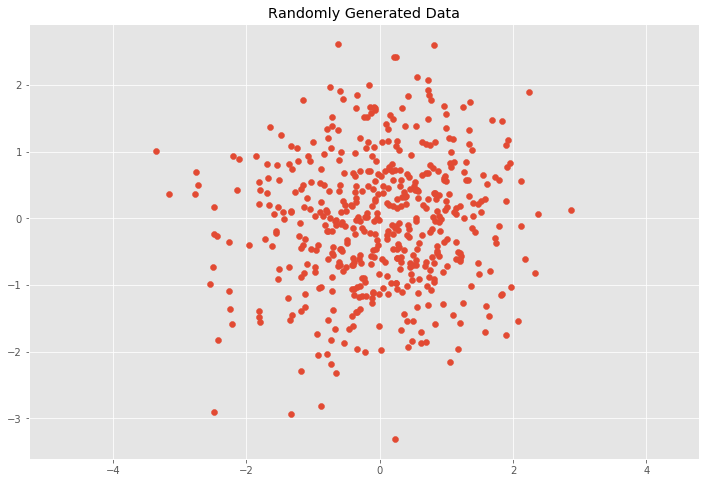This case would mean that x and y are independent (or uncorrelated) and the covariance matrix C is

$C=\left[\begin{array}{cc}{\sigma }_{x}^{2}& 0\\ 0& {\sigma }_{y}^{2}\end{array}\right]$

We can check this by calculating the covariance matrix as follows

In :
# Covariance
def cov(x, y):
xbar, ybar = x.mean(), y.mean()
return np.sum((x - xbar)*(y - ybar))/(len(x) - 1)

# Covariance matrix
def cov_mat(X):
return np.array([[cov(X, X), cov(X, X)], \
[cov(X, X), cov(X, X)]])

# Calculate covariance matrix
cov_mat(X.T) # (or with np.cov(X.T))

Out:
array([[1.06934588, 0.08151689],
[0.08151689, 0.98326751]])

The above results approximatelly gives us our expected covariance matrix with variances ${\sigma }_{x}^{2}={\sigma }_{y}^{2}=1$$\sigma_x^2=\sigma_y^2=1$.

## Linear Transformations of the Data Set¶

Now, we will see how linear transformations affect the data and the covariance matrix C. We will transform our data with the following scaling matrix.

$S=\left[\begin{array}{cc}{s}_{x}& 0\\ 0& {s}_{y}\end{array}\right]$

where the transformation simply scales the x and y components by multiplying them by ${s}_{x}$$s_x$ and ${s}_{y}$$s_y$ respectively. The covariance matrix C of the transformed data set will simply be

$C=\left[\begin{array}{cc}\left({s}_{x}{\sigma }_{x}{\right)}^{2}& 0\\ 0& \left({s}_{y}{\sigma }_{y}{\right)}^{2}\end{array}\right]$

which means that we can extract the scaling matrix from our covariance matrix by calculating $S=\sqrt{C}$$S=\sqrt C$ and the data is transformed by $Y=SX$$Y=SX$. .

In :
# Center the matrix at the origin
X = X - np.mean(X, 0)

# Scaling matrix
sx, sy = 0.7, 3.4
Scale = np.array([[sx, 0], [0, sy]])

# Apply scaling matrix to X
Y = X.dot(Scale)

plt.scatter(Y[:, 0], Y[:, 1])
plt.title('Transformed Data')
plt.axis('equal')

# Calculate covariance matrix
cov_mat(Y.T)

Out:
array([[ 0.52397948,  0.19401019],
[ 0.19401019, 11.36657236]])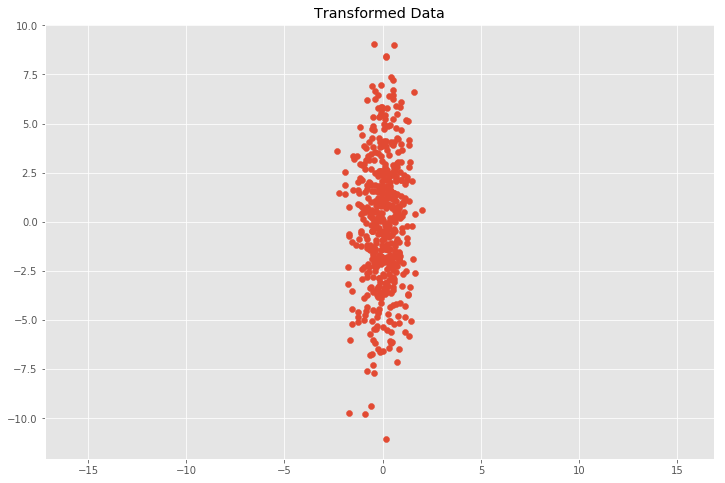Now we will apply a linear transformation in the form of a transformation matrix T to the data set which will be composed of a two dimensional rotation matrix R and the scaling matrix S as follows

$T=RS$

where the rotation matrix R is given by

$C=\left[\begin{array}{cc}cos\theta & -sin\theta \\ sin\theta & cos\theta \end{array}\right]$

where $\theta$$\theta$ is the angle of rotation. The transformed data is then calculated by Y = TX or Y = RSX.

In :
# Scaling matrix
sx, sy = 0.7, 3.4
Scale = np.array([[sx, 0], [0, sy]])

# Rotation matrix
theta = 0.77*np.pi
c, s = np.cos(theta), np.sin(theta)
Rot = np.array([[c, -s], [s, c]])

# Transformation matrix
T = Scale.dot(Rot)

# Apply transformation matrix to X
Y = X.dot(T)

plt.scatter(Y[:, 0], Y[:, 1])
plt.title('Transformed Data')
plt.axis('equal');

# Calculate covariance matrix
cov_mat(Y.T)

Out:
array([[ 5.07332695, -5.35423197],
[-5.35423197,  6.81722489]])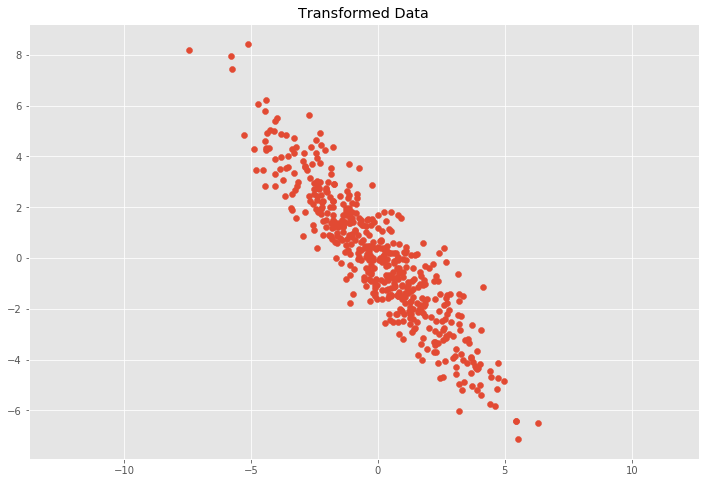### Eigen Decomposition of the Covariance Matrix¶

Eigen Decomposition is one connection between a linear transformation and the covariance matrix. An eigenvector is a vector whose direction remains unchanged when a linear transformation is applied to it. It can be expressed as

$Av=\lambda v$

where v is an eigenvector of A and $\lambda$$\lambda$ is the corresponding eigenvalue. If we put all eigenvectors into the columns of a Matrix $\mathbf{V}$$\bf V$ and all eigenvalues as the entries of a diagonal matrix L we can write for our covariance matrix C with the following equation

$CV=VL$

where the covariance matrix can be represented as

$C=VL{V}^{-1}$

which can be also obtained by $\bf Singular \ Value \ Decomposition$. The eigenvectors are unit vectors representing the direction of the largest variance of the data, while the eigenvalues represent the magnitude of this variance in the corresponding directions. This means V represents a rotation matrix and $\sqrt{L}$$\sqrt L$ represents a scaling matrix. From this equation, we can represent the covariance matrix C as

$C=RSS{R}^{-1}$

where the rotation matrix R = V and the scaling matrix $S=\sqrt{L}$$S=\sqrt L$. Hence,

$C=RSS{R}^{-1}=T{T}^{-1}$

because ${T}^{T}=\left(RS{\right)}^{T}={S}^{T}{R}^{T}=S{R}^{-1}$$T^T=(RS)^T=S^TR^T=SR^{-1}$ due to the properties ${R}^{-1}={R}^{T}$$R^{-1}=R^T$ since R is orthogonal and $S={S}^{T}$$S=S^T$ since S is a diagonal matrix. This enables us to calculate the covariance matrix from a linear transformation. In order to calculate the linear transformation of the covariance matrix, one must calculate the eigenvectors and eigenvectors from the covariance matrix C. This can be done by calculating

$T=V\sqrt{L}$

where V is the previous matrix where the columns are the eigenvectors of C and L is the previous diagonal matrix consisting of the corresponding eigenvalues. The transformation matrix can be also computed by the Cholesky decomposition with $Z={L}^{-1}\left(X-\overline{X}\right)$$Z = L^{-1}(X-\bar X)$ where L is the Cholesky factor of $C=L{L}^{T}$$C = LL^T$.

We can see the basis vectors of the transformation matrix by showing each eigenvector v multiplied by $\sigma =\sqrt{\lambda }$$\sigma = \sqrt \lambda$. By multiplying $\sigma$$\sigma$ with 3 we cover approximately 99.7% of the points according to the three sigma rule if we would draw an ellipse with the two basis vectors and count the points inside the ellipse.

In :
C = cov_mat(Y.T)
eVe, eVa = np.linalg.eig(C)

plt.scatter(Y[:, 0], Y[:, 1])
for e, v in zip(eVe, eVa.T):
plt.plot([0, 3*np.sqrt(e)*v], [0, 3*np.sqrt(e)*v], 'k-', lw=2)
plt.title('Transformed Data')
plt.axis('equal');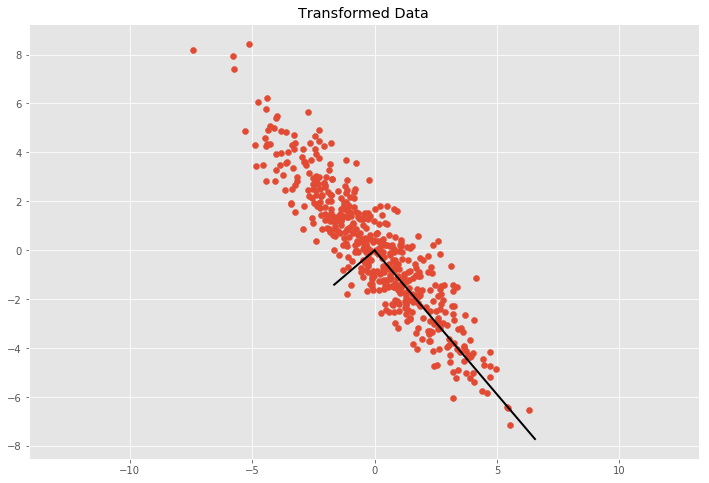We can now get from the covariance the transformation matrix T and we can use the inverse of T to remove correlation (whiten) the data.

In :
C = cov_mat(Y.T)

# Calculate eigenvalues
eVa, eVe = np.linalg.eig(C)

# Calculate transformation matrix from eigen decomposition
R, S = eVe, np.diag(np.sqrt(eVa))
T = R.dot(S).T

# Transform data with inverse transformation matrix T^-1
Z = Y.dot(np.linalg.inv(T))

plt.scatter(Z[:, 0], Z[:, 1])
plt.title('Uncorrelated Data')
plt.axis('equal');

# Covariance matrix of the uncorrelated data
cov_mat(Z.T)

Out:
array([[1.00000e+00, 1.06795e-16],
[1.06795e-16, 1.00000e+00]])